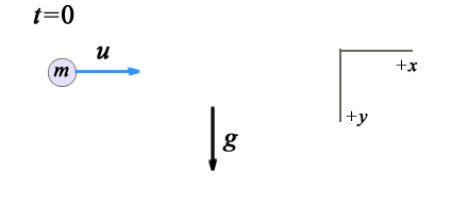# Direction of acceleration of a projectile with drag force

baseballfan_ny
Homework Statement:
At low speeds (especially in liquids rather than gases), the drag force is proportional to
~ the velocity, i.e., F = −C ~v, where C is a constant. At time t = 0, a small ball of mass
m is projected into a liquid so that it initially has a horizontal velocity of magnitude
u in the +x direction as shown. (The vertical component of the velocity is zero). The
gravitational acceleration is g. Consider the cartesian coordinate system shown in the
figure (+x to the right and +y downwards).

(a) What is the component of the acceleration in the x direction for t > 0? Express
your answer in terms of vx (the component of the velocity in the x direction), C,
g, m and u as needed.
Relevant Equations:
F = -bv
F = ma
a = dv/dtFirst and foremost, thank you so much for your help! I'm new to Physics Forums, and this is my first post.

I thought I understood the problem, but as I moved on to the subsequent parts of it became apparent that I was missing something. I set up my ##F = ma## equation as below, and solved that for ##a_x##.
$$\sum F_x = -Cv(t)_x = m(-a_x)$$
$$a_x = \frac {C*v(t)_x} {m}$$

However, I think this is incorrect, but I just can't conceptualize why. The resistive force on the mass points in the ##-\hat x## direction and the mass starts with an initial velocity directed in the ##+\hat x## direction. I assumed that the acceleration of the mass must also point in the ##-\hat x## direction to oppose the velocity and slow the mass down. But this doesn't seem to be right, as it would imply an infinite terminal velocity for the mass and most online solutions seem to show that
$$\sum F_x = -Cv(t)_x = m(a_x)$$

I just don't get why the acceleration points in the opposite direction of the resistive force. If this resistive force is the only force on the mass, shouldn't the acceleration have the same direction? And doesn't the acceleration need to be in the opposite direction of the initial velocity to slow the mass down?

Homework Helper
Gold Member
2022 Award
$$\sum F_x = -Cv(t)_x = m(-a_x)$$
According to master Newton
$$\sum F_x = m(a_x)$$

•etotheipi
baseballfan_ny
Thanks for the response!

I guess it makes sense that to follow Newton's 2nd Law, ##\sum F_x = m(a_x)##. But I'm still a little bit confused on the mathematical interpretation. Shouldn't the acceleration point in the direction opposite the initial velocity to slow the mass down?

Thanks!

Homework Helper
Gold Member
2022 Award
Shouldn't the acceleration point in the direction opposite the initial velocity to slow the mass down?
With the corrected sign it does:
##-Cv(t)_x=\sum F_x = m(a_x)##
##a_x=-\frac{Cv(t)_x}m##

•baseballfan_ny and etotheipi
baseballfan_ny
Oh! I think I get it now. ##a_x## is not a vector but a component, and it's equal to the sum of the left hand side divided by m. So the actual vector ##\vec a## would be something like ##\vec a## = <-##\frac{Cv(t)_x}m##, g>, which clearly shows that the horizontal component of the acceleration points opposite the velocity.

•